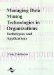# Alternative Weighting Methods

 < Day Day Up >

The identification of the forecasting methods stage then left 24 out-of-sample forecasts for weights estimation and comparisons. Four alternative weighting methods are compared.

1. Simple Average (SA). A simple linear average of the forecasts, implying equal weights.

2. Fixed Weighting (FW). Weights are estimated on the first 12 out-of-sample forecasts and these weights are then used unchanged to combine forecasts for the next 12 periods.

3. Rolling Window Weighting (RW). A rolling forecast window of 12 periods is employed. Initially, the model is run with the 12 out-of-sample forecasts for periods 37 to 48 to estimate the weights to make the combined forecast for the 49th period (which is the same as for the Fixed Weights case). The quadratic programming model is then rerun with out-of-sample forecasts for periods 38 to 49 to determine the best weights, which are then used to give the combined forecast for the 50th period. This process is continued to give combined forecasts for periods 51 to 60.

4. Highest Weighting (HW). The individual forecast procedure that was given the highest weight in the Fixed Weighting method. This is not a combination. This method is equivalent to choosing the forecasting technique which is the best on the weight estimation period.

The above procedure was adopted so that the alternative methods can be compared on their forecasting performance on exactly the same data set of demands. All the forecasts are one-step-ahead so the comparisons are on a one-step-ahead basis. In the situation of this research, this is what is required because stocks are reviewed every month. The individual forecasting models identified and fitted over periods 1 to 36 were not updated thereafter over periods 37 to 59.

 < Day Day Up >Managing Data Mining Technologies in Organizations: Techniques and Applications
ISBN: 1591400570
EAN: 2147483647
Year: 2003
Pages: 174